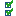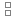The Rocscience International Conference 2021 Proceedings are now available. Read Now

# Joint Spacing

The Joint Spacing option allows you to calculate the true joint spacing of joints in a joint Set, calculated from distance measurements recorded along a linear or borehole Traverse.

In order to use the Joint Spacing option:

1. You must have at least one (or more) joint Sets defined, using the options available for creating Sets (e.g. Add Set Window, Add Set Freehand).
2. You must have at least one (or more) Linear or Borehole Traverses defined (e.g., Linear, Linear BH Oriented Core, Linear BH Televiewer, Curved BH Oriented Core, Curved BH Televiewer).
3. The Distance Column must be enabled (checkbox in Project Settings) and Distance values entered in Grid Data view.
4. If the above 3 criteria are met:

5. Select Joint Spacing from the Analysis menu.
6. In the Joint Spacing dialog:
• Select the Spacing from either True Spacing or Apparent Spacing
• Select a joint Set.
• Select all or any combination of individual Traverse(s). You can quickly select all or no Traverses using the Select All/ Deselect Alloptions.

NOTE: At least one Traverse must be selected.
7. Select OK and a distribution graph of joint spacing (true or apparent) and statistics will be generated for the selected joint set and Traverse(s). See below for more details.

## True Spacing and Apparent Spacing

The Apparent Spacing is equal to the difference in Distance values (entered in the Distance column) between adjacent joints which belong to the SAME joint set. This is the raw unprocessed spacing as measured directly from a linear Traverse, borehole or oriented core.

The True Spacing is the actual perpendicular spacing between adjacent joints in a joint set. The True Spacing is always less than or equal to the apparent spacing, and is calculated as follows:

True Spacing = Apparent Spacing * cos ( a) = Apparent Spacing / Terzaghi Weighting

where a = the angle between the pole of the mean joint set plane and the linear Traverse direction; alternatively, Terzaghi Weighting (= 1 / cos ( a)) = the bias correction factor for the mean joint set plane and the linear Traverse direction. If joints are exactly perpendicular to a linear Traverse then true spacing = apparent spacing.

## Joint Set

You can only choose one joint Set for plotting at a time. To analyze spacing for a different joint Set, repeat the above steps for each desired Set.

## Traverses

You can choose to plot spacing data based on All Traverses together, or select any combination of individual traverses.

NOTE: The Spacing calculation is valid for all Traverse types except Planar, since the calculation assumes data collection along a line rather than a planar surface.

## Summary of Spacing Results

The Info Viewer automatically provides a summary of joint spacing results for all Sets and all Traverses, as long as the 3 criteria are met (Linear Traverses / Sets / Distance column). Even if you do not actually select the Joint Spacing option to plot results, the Joint Spacing summary will be available in the Info Viewer.